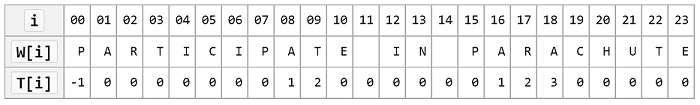# Searches and Matching

#### sal/algo/search.h

Suffix_array efficient data structure for indexing large texts bin_search binary search on ordered sequence intersection set of common elements among a set of sets select order selection of ith smallest element from unsorted sequence sub_match search for occurance of a substring inside a larger string lc_subseq longest common subsequence between 2 sequences lc_subseq_len length of longest common subsequence between 2 sequences lc_substr longest common substring using a Suffix array

### Binary search ¶

Declaration

Parameters

 begin iterator pointing to the first sequence element end iterator pointing to one past the last sequence element key value to find; should be an argument to `cmp.eq` and `cmp.less` cmp comparator that should supply `bool less(T,T)` and `bool eq(T, T)`

Example

Discussion

A fundamental algorithm used because of its `Θ(lg(n))` time complexity (n is sequence length). This is in comparsion to linear sort, which has `Θ(n)` time complexity. As expected, this lg(n) performance is due to reducing the problem size to half each run through the Divide and Conquer strategy. Each iteration compares the median value against the target, relying on the sorted property to eliminate the half that is on the opposite side of the target relative to the median.

Some pitfalls to binary search are 1. it requires random access to the entire sequence, which implies

1. the entire sequence need to be loaded in memory (or disk access will slow it down dramatically), and for
likely be more apparent in the future when more advanced optimizations for space locality becomes implemented.

### Intersection ¶

Declaration

Parameters

 s set of sequences from which the intersection should be taken on

Example

Discussion

Optimal with time complexity `O(n)` (n is total sum of sequence elements). In the worst case, each element will be looked at if all elements are shared. This algorithm, like many other element matching ones, is based on hashtable lookups, which give average `O(1)` complexity. For known value ranges that's uniquely mappable to natural numbers, such as language alphabet, a fixed size array could be used with the value's number value as the hash.

For example, the UTF-8 encoding represents characters with 8 bits, uniquely mapping each character to a number between 0 and 255. An array of size 256 could be used to find the intersection and other characteristics among UTF-8 sequences.

### Order selection ¶

Declaration

Parameters

 begin iterator pointing to the first sequence element end iterator pointing to one past the last sequence element i ith smallest element of a sequence to select (1 is smallest)

Example

Discussion

Selecting for minimums and maximums are relatively easy tasks requiring `O(n)` time, but finding an arbitrary ith ordered element requires a different approach.

The algorithm implemented is quickselect, which, as the name suggests, is very similar to quicksort with `O(n)` performance. Their approach is the same - choosing a pivot to partition the sequence around based on <, but unlike quicksort, which recurses on both sides, quickselect recurses only on the side the target is on (found by comparing the rank=(pivot-begin) of the pivot against i).

At the end of selection, the sequence will be partially sorted with the ith element being the ith smallest, and everything smaller than it on its left while everything larger than it on the right, with no order guranteed.

### Substring matching ¶

Declaration

Parameters

 s an indexable sequence; the larger one - the "sentence" w the same type of sequence; the smaller one - the "word"

Example

Discussion

An `O(n)` (n is length of sentence) algorithm for finding a substring inside a larger sentence. This finds applications in biomedical computing, such as finding a codon sequence inside a chromosome.

This algorithm uses the information implicit in where the matching failed inside the word to prevent wasteful backtracking. An example from wikipedia:When matching fails before 'A' with i=8, there is no possibility that another match started during the match, as the word starts with 'P' and none have been encountered. For backtracking to be necessary, the matching has to fail on a prefix of the word, such as "PA-" at i=9, and "PAR-" at i=18.

### Longest common subsequence ¶

Declaration

Parameters

 a An indexable sequence b Another sequence of the same type

Example

Discussion

A subsequence is made by removing some elements but keeping the same order. A substring in addition needs to be from continuous elements. Finding the longest common subsequence (LCS) is crucial for resolving similarity among data, important for data compression, revision control, and bioinformatics.

A dynamic programming approach is used since the LCS of the two is also the LCS-last_matched_char of the two sequences less their last matched character. This yields `O(n*m)` time and space performance (n is length of a, m is length of b) for retrieving the LCS, and only `O(min(n,m))` space for retrieving the LCS length.

### Longest common substring ¶

Declaration

Example

Discussion

Using a suffix array, the longest common substring can be found in `O(n)` time (although SAL's implemenation of a suffix array renders it `O(n(lg(n))^2)` time. This is done by concatenating the two sequences and indexing it into a suffix array. Then the longest common prefix (LCP) array is iterated over to find the maximum value filtering out the substrings from the same initial sequence. This algorithm can be extended to an arbitrary number of sequences, with the only alteration being that the scan over the LCP array needs to filter on consecutive n-suffixes sharing a minimum prefix, as well as additional checks for whether all initial sequences have been covered.